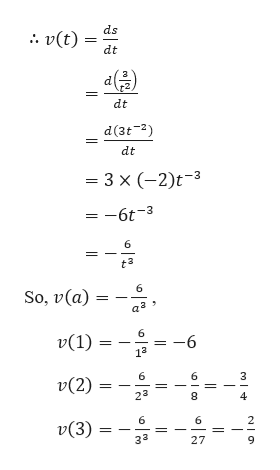# The displacement (in meters) of a particle moving in a straight line is given by the equation of motion s = 3/t2,where t is measured in seconds. Find the velocity of the particle at times t = a, t = 1, t = 2, and t = 3.

Question

The displacement (in meters) of a particle moving in a straight line is given by the equation of motion

s = 3/t2,

where t is measured in seconds. Find the velocity of the particle at times

t = a, t = 1, t = 2, and t = 3.
check_circleExpert Solution
Step 1

Given,

Step 2

The velocity is the de...help_outlineImage Transcriptioncloseds v(t) dt dt d(3t2 dt - 3 x (-2)t3 = -6t-3 6 6 So, v(a) 6 v(1) -6 13 6 6 3 v(2) 23 8 4 2 v(3) 32 27 미인 fullscreen

### Want to see the full answer?

See Solution

#### Want to see this answer and more?

Solutions are written by subject experts who are available 24/7. Questions are typically answered within 1 hour*

See Solution
*Response times may vary by subject and question
Tagged in

### Derivative